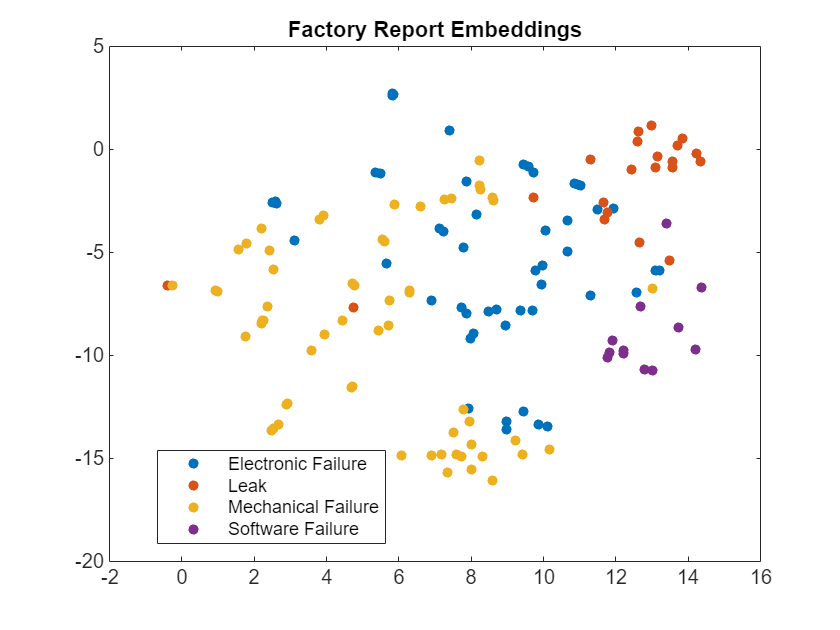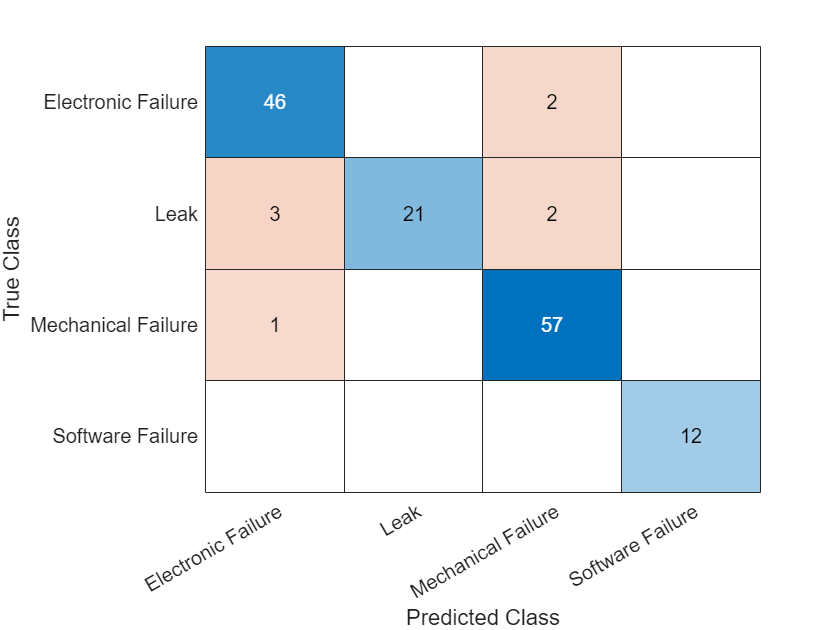# Classify Documents Using Document Embeddings

This example shows how to train a document classifier by converting documents to feature vectors using word embeddings.

Most machine learning techniques require feature vectors as input to train a classifier.

A word embedding maps individual words to vectors. You can use a word embedding to map a document to a single vector by combining the word vectors, for example, by calculating the mean vector to create a document vector.

Given a data set of labeled document vectors, you can then train a machine learning model to classify these documents.

Load a pretrained word embedding using the `fastTextWordEmbedding` function. This function requires Text Analytics Toolbox™ Model for fastText English 16 Billion Token Word Embedding support package. If this support package is not installed, then the function provides a download link.

`emb = fastTextWordEmbedding`
```emb = wordEmbedding with properties: Dimension: 300 Vocabulary: ["," "the" "." "and" "of" "to" "in" "a" """ ":" ")" "that" "(" "is" "for" "on" "*" "with" "as" "it" "The" "or" "was" "'" "'s" "by" "from" "at" … ] ```

For reproducibility, use the `rng` function with the `"default"` option.

`rng("default");`

The next step is loading the example data. The file `factoryReports.csv` contains factory reports, including a text description and categorical labels for each event.

```filename = "factoryReports.csv"; data = readtable(filename,TextType="string"); head(data)```
```ans=8×5 table Description Category Urgency Resolution Cost _____________________________________________________________________ ____________________ ________ ____________________ _____ "Items are occasionally getting stuck in the scanner spools." "Mechanical Failure" "Medium" "Readjust Machine" 45 "Loud rattling and banging sounds are coming from assembler pistons." "Mechanical Failure" "Medium" "Readjust Machine" 35 "There are cuts to the power when starting the plant." "Electronic Failure" "High" "Full Replacement" 16200 "Fried capacitors in the assembler." "Electronic Failure" "High" "Replace Components" 352 "Mixer tripped the fuses." "Electronic Failure" "Low" "Add to Watch List" 55 "Burst pipe in the constructing agent is spraying coolant." "Leak" "High" "Replace Components" 371 "A fuse is blown in the mixer." "Electronic Failure" "Low" "Replace Components" 441 "Things continue to tumble off of the belt." "Mechanical Failure" "Low" "Readjust Machine" 38 ```

The goal of this example is to classify events by the label in the `Category` column. To divide the data into classes, convert these labels to categorical.

`data.Category = categorical(data.Category);`

The next step is to create a partition to split our data into sets for training and testing. Partition the data into a training partition and a held-out partition for validation and testing. Specify the holdout percentage to be 30%.

`cvp = cvpartition(data.Category,Holdout=0.3);`

Use the partitions to obtain the target labels for training and test. Later in the example, after the creation of the vectors for the documents, the partition will also be used to split the input data into training and test.

```TTrain = data.Category(training(cvp),:); TTest = data.Category(test(cvp),:);```

It can be useful to create a function that performs preprocessing so you can prepare different collections of text data in the same way.

Create a function that tokenizes and preprocesses the text data so it can be used for analysis. The `preprocessText` function, listed in the Example Preprocessing Function section of the example, performs the following steps:

1. Tokenize the text using `tokenizedDocument`.

2. Erase punctuation using `erasePunctuation`.

3. Remove a list of stop words (such as "and", "of", and "the") using `removeStopWords`.

4. Lowercase all words.

`documents = preprocessText(data.Description);`

### Convert Documents to Feature Vectors

The function `word2vec` is used to convert from tokens to vectors. For larger documents, it's possible to combine these word vectors into a single one computing the mean across all words. This example shows how to obtain document vectors.

Obtain the vector for each document computing the mean across all the words in the document. Compute the word vectors using the `word2vec` function and remove any words missing from the embedding vocabulary using the `rmissing` function. Calculate the mean over the document's words (the first dimension of the data).

```meanEmbedding = zeros(numel(documents),emb.Dimension); for k=1:numel(documents) words = string(documents(k)); wordVectors = word2vec(emb,words); wordVectors = rmmissing(wordVectors); meanEmbedding(k,:) = mean(wordVectors,1); end meanEmbeddingTrain = meanEmbedding(training(cvp),:); meanEmbeddingTest = meanEmbedding(test(cvp),:); ```

View the size of the embedded test data. The array is a numObservations-by-embeddingDimension array where numObservations is the number of test documents, and embeddingDimension is the embedding dimension.

`size(meanEmbeddingTest)`
```ans = 1×2 144 300 ```

The output for each document is a single 300 dimension array that summarizes all the features of the word vectors contained in the document. The vector for the first document in the test set is obtained as follows:

`meanEmbeddingTest(1,:)`
```ans = 1×300 -0.1367 -0.0284 -0.1061 -0.0034 0.0577 -0.0662 -0.0845 -0.0606 0.0117 -0.0614 0.1074 -0.0814 0.0160 -0.0101 -0.0419 -0.0108 -0.0433 -0.0334 -0.0192 -0.0640 -0.1802 -0.0926 0.0291 -0.0787 0.1210 -0.0796 0.1160 -0.0278 -0.0243 -0.0577 0.0851 0.0354 0.0002 0.0060 0.0887 0.0491 0.0312 -0.0865 -0.0867 0.0378 -0.0794 -0.1174 0.0331 0.0432 0.0372 -0.0873 -0.0050 -0.0515 0.0382 0.0283 ```

Once the document vectors are obtained, it's also possible to embed the document vectors in a two-dimensional space using `tsne` by specifying the number of dimensions to be two. A t-SNE plot can help show clusters in the data, which can indicate that you can build a machine learning model.

```Y = tsne(meanEmbeddingTest); gscatter(Y(:,1),Y(:,2), categorical(TTest)) title("Factory Report Embeddings")```### Train Document Classifier

After visualizing the document vectors and respective clusters, you can train a multiclass linear classification model using `fitcecoc`.` `

`mdl = fitcecoc(meanEmbeddingTrain,TTrain,Learners="linear")`
```mdl = CompactClassificationECOC ResponseName: 'Y' ClassNames: [Electronic Failure Leak Mechanical Failure Software Failure] ScoreTransform: 'none' BinaryLearners: {6×1 cell} CodingMatrix: [4×6 double] Properties, Methods ```

### Test Model

Compute the scores for the mean vectors, visualizing the accuracy results and the confusion matrix.

```YTest = predict(mdl,meanEmbeddingTest); acc = mean(YTest == TTest)```
```acc = 0.9444 ```
`confusionchart(YTest,TTest)`Large numbers on diagonal indicate good prediction accuracy for the corresponding classes. Large numbers on the off-diagonal indicate strong confusion between the corresponding classes.

### Example Preprocessing Function

The function `preprocessText`, performs the following steps in order:

1. Tokenize the text using `tokenizedDocument`.

2. Erase punctuation using `erasePunctuation`.

3. Remove a list of stop words (such as "and", "of", and "the") using `removeStopWords`.

4. Lowercase all words.

```function documents = preprocessText(textData) % Tokenize the text. documents = tokenizedDocument(textData); % Erase punctuation. documents = erasePunctuation(documents); % Remove a list of stop words. documents = removeStopWords(documents); % Lowercase all words. documents = lower(documents); end```